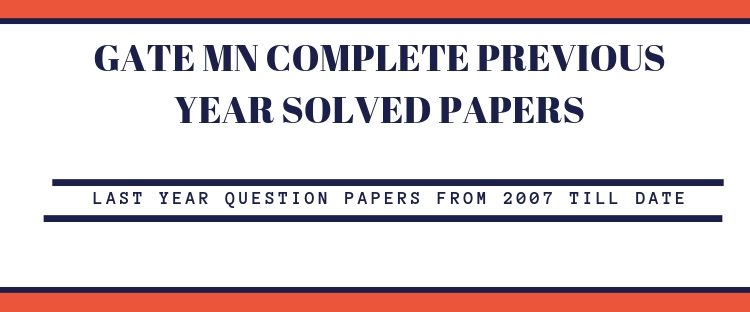Previous 12 Year Papers and Keys of GATE-MN

GATE Mining Engineering (MN) Previous Question papers with answersGraduate Aptitude Test in Engineering (GATE) is an examination held in February by both the Indian Institute of Science (IISc), Bangalore and seven Indian Institutes of Technology on behalf of MHRD and Government of India to test the knowledge of the candidates in various undergraduate subjects related to Science. GATE Qualification is mandatory for the students seeking admission to Master’s programs like ME, M. Arch and PH.D. programs in relevant branches of Science, in the institutions supported by the MHRD and other Government agencies.

GATE Exam Pattern

The GATE Exam is for a duration of 3 hours and the pattern of the Question Paper is same as the other branches of GATE examination. The Question paper has 65 questions of 1 mark and 2-mark each totaling to 100 marks for the entire paper. The pattern of the Question Paper is same as the other branches of GATE examination.

The question paper consists of 2 types of questions:
Multiple Choice Questions (MCQ) and

• Multiple Choice Questions (MCQ) – Each MCQ would carry 1 or 2 marks and they are objective in nature, and each will have a choice of four answers, out of which the candidate must choose the correct answer. Note that there will be negative marking for a wrongly selected answer.
• Numerical Answer Type (NAT) Questions -Each NAT question carry 1 or 2 marks and for these questions, the answer is a real number and no choices are provided. There is no negative marking for a wrong answer in NAT questions.

The score gained for GATE Examination is accepted in more than 900+ institutes across India and is valid for three years post results.

GATE MN Syllabus:

• Section 1: Engineering Mathematics

Linear Algebra: Matrices and determinants, Eigen values and Eigen vectors.

Calculus: Partial derivatives; maxima and minima; Fourier series, Taylor series.

Vector Calculus: Gradient; divergence and curl; line; surface and volume integrals; Stokes, Gauss and Greens theorems.

Differential Equations: Cauchy’s and Euler’s equations; Laplace transforms; Partial Differential Equations – Laplace, Linear and non-linear first order Ordinary Differential Equations (ODE); Higher order linear ODEs with constant coefficients.

Probability and Statistics: Poisson, normal and binomial distributions; correlation and regression analysis.

Numerical Methods: Solutions of linear and non-linear algebraic equations; numerical integration – trapezoidal and Simpson’s rule.

• Section 2: Mine Development and Surveying

Mine Development: Methods of access to deposits; Underground drivages; Drilling methods and machines.

Mine Surveying: Levels and leveling, theodolite, tacheometry, triangulation; Photogrammetry; Field astronomy; EDM and Total Station; Introductory GPS.

• Section 3: Geomechanics and Ground Control

Engineering Mechanics: Equivalent force systems; Free body diagrams; Friction forces; Particle kinematics and dynamics; Beam analysis.

Geomechanics: Geo-technical properties of rocks; Rock mass classification; Theories of rock failure; Ground vibrations; Subsidence; Rock bursts and coal bumps; Slope stability.

Ground Control: Design of pillars; Roof supporting systems; Mine filling.

• Section 4: Mining Methods and Machinery

Mining Methods: Surface mining: layout, development, transportation and mechanization, continuous surface mining systems; stope mechanization, ore handling systems.

Mining Machinery: Generation and transmission of mechanical, hydraulic and pneumatic power; Materials handling.

• Section 5: Surface Environment, Mine Ventilation, and Underground Hazards

Surface Environment: Air, water and soil pollution; Noise; Land reclamation.

Mine Ventilation: Underground atmosphere; Mine fans and their usage; Auxiliary ventilation; Ventilation planning.

Subsurface Hazards: Rescue apparatus and practices; Safety in mines; Accident data analysis; Mine lighting; Mine legislation; Occupational safety.

Section 6: Mine Economics, Mine Planning, Systems Engineering

Mine Economics – Discounted cash flow analysis; Mine valuation; Mine investment analysis;

Mine Planning – Reserve estimation techniques: Basics of geo-statistics and quality control; Optimization of facility location; Work-study.

Systems Engineering:  Linear programming and assignment problems; Network analysis; Inventory models; Queueing theory; Basics of simulation.

Click on GATE MN Syllbus for 2020 to get the detailed syllabus of GATE 2020 Mining Engineering (MN).

GATE MN Complete Previous Year Question Papers and Answer Keys: